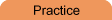Home    |    Teacher    |    Parents    |    Glossary    |    About UsEmail this page to a friendSelect Subject-Select Subject Basic Math Everyday Math Pre-Algebra Algebra Geometry Trigonometry Statistics Calculus Advanced Topics OthersResourcesCool Tools Formulas References Study Tips Wonders of MathSearchAlgebra Worksheet Generator
 Teacher Name: Worksheet Title: Select number of each type of equations: One-step Equations: (e.g.. x-4=10) Two-step Equations: (e.g.. 2x+6=16) Combining Like Terms X's on both sides Distributive Property Systems - solve for x and y solve by adding solve by subtracting a mix of the above two require one multiplication step general system solve by substitution Quadratics - ax2+bx+c=0 simple factorable difficult factorable (a not equal 1) general - require quadratic formula imaginary solutions, general Note: There will be no ads on the worksheet. Options: Create Answer Sheet also Include Header No Negatives 0 5 10 20 30 40 50 60 70 80 90 100 Percent of coefficients that are fractions Printing tips - For best printed results. Permissions - Can you photocopy these worksheets? Answer sheet pops up in separate window. Please disable popup blocker or try using Internet Explorer if it doesn't appear.